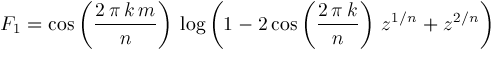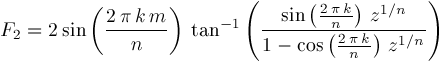ISSAC 96 - Hypergeometric Function Representations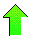Contents News View

 Lerch Phi and Polylogarithms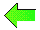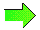If the coefficients of the series representation of a hypergeometric function are rational functions of the summation index, then the hypergeometric function can be expressed as a linear sum of Lerch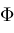functions.  The Lerchfunction is defined by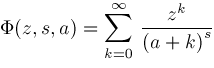Further, if the parameters of the hypergeometric function are rational, we can proceed to express the hypergeometric function as a linear sum of polylogarithms.  The polylogarithm function is defined by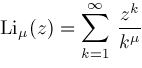The first theorem shows how to express such a hypergeometric function as a linear sum of Lerchfunctions.

Theorem Let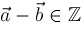,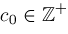, and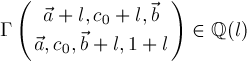have partial fraction decomposition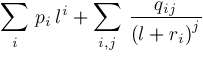Then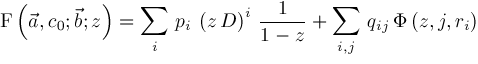The next theorem can be used to range reduce the third argument of a Lerchinto the interval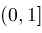Theorem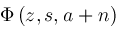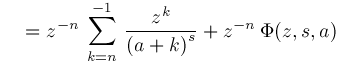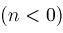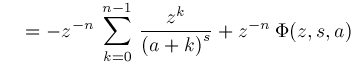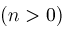The next two theorems show how to convert Lerchinto polylogarithms if the third argument is rational.

Theorem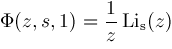Theorem Let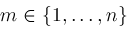and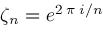.  Then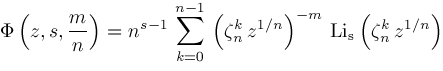Corollary Letand.  Then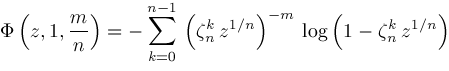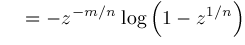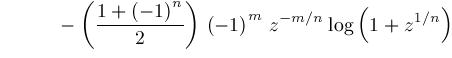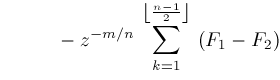where